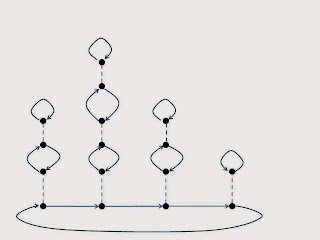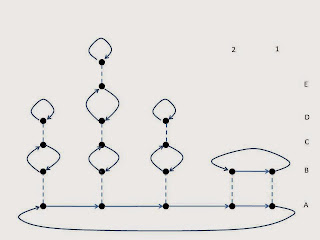## Saturday, September 6, 2014

### Writing on a Turing Tape

In an earlier post here, Computing with Graphs, I proposed a class of graphs and a few simple rewrite rules that could provide a way to store computational data. These graphs could provide a more realistic memory model than either Turing tapes or random access memories. In that post I sketched a proof that the rewrite rules were complete, i.e. they provide a path from any graph in the proposed class to any other graph in the class.

Now I would like to provide the basis for a proof of Turing completeness. If a Turing machine simulator can be built using this class of graphs, then the memory model is at at least minimally adequate as a computational storage medium. I will just outline one way to use the graphs to represent simple Turing tapes.

The tape itself is a sequence of graph nodes connected by directed arrows. The tape is always finite in length but can grow indefinitely in either direction, so it can simulate an infinite tape. Each node connects via an undirected edge to the data for that node. The data contained on a cell of a Turing tape is a symbol drawn from some finite set. Using the graphs, each symbol is represented by a subgraph of some unique shape, restricted only in having a single outgoing undirected edge by which is is connected to the cell's tape node. One special subgraph represents the end of the tape. Whenever the head bumps up against that special marker, the tape can be extended by adding another blank symbol.

So here is a graph representing a Turing tape with three cells. The fourth tape node is the one that contains the tape end marker:I will show the steps involved in extended the tape with a new blank symbol. First, an expansion rewrite operation is performed on the undirected edge that connects the tape end symbol to its tape node:I have added some location marks to facilitate the identification of edges. Next, the outgoing edges of the undirected edge A2-B2 are swapped:

Then an undirected edge swap is performed on the directed edge A2-B1:

An outgoing edge swap on undirected edge B1-B2:

An undirected edge swap on directed edge B2-A1:

At this point it seems that a new operation is required, where the incoming directed edges of an undirected edge are swapped. The proof sketch of my earlier post would seem to imply that this operation is unnecessary. In any case it seems very natural and convenient at this point.

So, then, an incoming edge swap on undirected edge A1-B1:

An expansion on undirected edge A2:B2:

An outgoing edge swap on undirected edge A2-B2:

An undirected edge swap on directed edge E2-B2:

An outgoing edge swap on undirected edge A2-E2:

An outgoing edge swap on undirected edge B2-D2:

An undirected edge swap on directed edge A2-B2:

An outgoing edge swap on undirected edge A2-D2:

An undirected edge swap on directed edge D2-B2:

An outgoing edge swap on undirected edge A2-B2:

One approach to a representing a finite set of symbols of arbitrary size is just to create strings of loops of arbitrary height. The steps above first created an extra end-of-tape symbol and then expanded it to a blank symbol. The steps that expanded the end-of-tape to the blank could be repeated on the blank to create the first non-blank symbol, and from there repeated as many times as necessary to create whatever symbol is required out of the finite set of symbols. These operations can be performed in reverse, so the replacement of one symbol by another is straightforward.